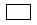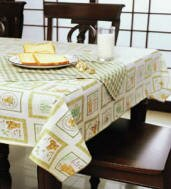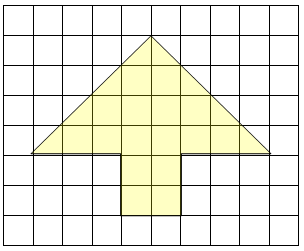Interactive Math Virtual Manipulatives Pattern Blocks Meters-Liters-Grams Reading Practice

More Area and Perimeter

Carefully examine each of the three rectangles shown below.

 A B C D= 1 square cm

Calculate the perimeter and the area of each rectangle above. Label each answer.

 RECTANGLE PERIMETER AREA A B C D

And Even More Area and Perimeter Practice

Examine rectangles A, B, and C below.
Calculate the perimeter and the area of each one.

 6 ft. A 5 in. B 7ft. 2 in. C 25 in.
 RECTANGLE PERIMETER AREA A B C

Covering Box Tops with Paper
The pink and orange rectangles below are the tops of gift boxes. You want to cover each of them with fancy paper. How much paper will you need to cover each one?

 33 cm 2 cm 12 cm 5 cm

To cover the pink rectangle you need
To cover the orange rectangle you need

Thanksgiving DinnerAunt Mary is preparing her table for Thanksgiving dinner. She wants her tablecloth to hang 6 inches over all sides of the tabletop. The table measures 60 inches long and 30 inches wide. What is the area of the tablecloth that Aunt Mary needs?

Open Response Questioning Strategies

 Templates for Constructing Steps to Writing Open-Ended Questions 4-Column method of Answering Open Response Examples of Open-Ended Questions

Templates for Constructing Open- Ended Questions:

 Analysis
 What are some possible explanations as to why_____________?
 Would other ________be affected by __________? Why or why not?
 Tell what ____________did wrong. How would you design a better way?
 Draw a describe __________. Explain why ____________.
 Explain several reasons why ___________.
 Explain how ______________.
 Describe several ways __________________.
 Describe several things we can do to _____________________.
 Discuss (describe) _____________. Explain how ____________.
 Explain how you arrived at your answer using pictures, words, equations. (Math)
 What is the fewest number ______________? (Math)
 What is the most ________________? (Math)
 Discuss the likelihood that _______________.
 From the information on chart, what is true of ________________?
 List the property of ____________. How are ____________?
 Show or explain the role of ______________. (how __________).
 Would everyone agree with ___________? Why or why not?
 Comparison & Problem Solving
 Suppose you want to __________. Make a _______ and ____________. Tell why you chose (included) each.
 Suppose you were a __________. Tell how you ________. Explain why you _________.
 If ___________, how would ___________be affected. (why)?
 Make a graph showing ____________. (Math)
 Would you rather have __________ or ___________? Tell why. (Math)
 Discuss the pros and cons of _______________.
 Discuss differences and similarities that exist between ______________. Include advantages and disadvantages.
 Think of a problem that ____________. Describe the problem and give a solution.
 Compare the ____________. Tell which ____________would be most likely and which _______would be least likely. Support your answer.
 Tell what would happen if ____________.
 List the differences (similarities) in _____________.
 What are the advantages of __________? What are the disadvantages of __________? What would you do? Why?
 Evaluation
 Imagine you are _____________ (in _____________).
 A. Based on what you know about __________, select (chose/decide) ________ and explain your selection (chose/decision).
 B. Write an argument you would use to convince_____________. Use information from your reading to support your argument.
 Would you like to ___________? Why or why not? Explain your answer giving examples from__________.

Steps to Writing Open-Ended Questions:

 1) Examine your subject or topic.
 A. Decide on concepts that could be assessed using open-ended questions.
 B. Make a list of three or four ideas.
 C. Plan the purpose and decide what part of your lesson would be best for using open-ended questions.
 2) Decide what you want students to do.
 A. Consider many different possibilities to determine the best format to use. (Do you want students to compare, contrast, to describe, to evaluate, to predict, etc.?)
 B. Decide if there is a match between the content and the expectations you have for students.
 3) Use the RAMPS procedure for constructing the prompt.
 A. Write a scenario for the prompt. (a description of the situation).
 1. What is the student's role? (R)
 2. Is there a particular audience? (A)
 3. What is the context (setting) for the problem? (S) Other considerations (optional):
 4. What is the problem?
 5. What assumptions (if any) should be included?
 B. Write the expectations related to students responding to the question.
 1. Decide the mode (or form) that students are to use (i.e. a letter, a plan, a summary, explanation, etc.) (M)
 2. Decide the purpose (i.e. to evaluate, compare, explain, predict, etc.) (P) Other considerations.
 3. Make sure expectations are specific.
 4. Include any specific content/concepts you want the student to explain.
 5. Decide if students should include representations such as charts, diagrams, or pictures with their explanations.
 4) Develop a scoring guide.

Four Column Method for Answering Open-Ended Questions:

 In tracking OR questions, students use the 4 column method to read the question critically, think through a plan, and write an outline for an answer.
 Columns 1 & 2 require reading Column 3 requires integration of process and content Column 4 makes connections and moves students beyond proficiency

Four Column Method Continued:

 Students will need multiple experiences with the 4 column method to develop proficiency with the need skills. Teachers can provide those experiences by following the suggested process below:
 1-Students work in triads (groups of 3) to analyze an OR question using the 4 column method and then write a proficient response. Using existing KIRIS questions and their rubrics allows students to see immediately if their group response was proficient.
 2-Students continue to work in triads, responding to cued OR prompts (questions that relate to material recently covered in the class). They complete the four column model together and write an answer together. Students remain at this step until, as a group, they can write proficient responses.
 3-Students respond individually to cued OR prompts on an individual basis, using the four column model. With completed answers, the triad members share their responses and assist in reshaping answers to improve quality. This step would also be repeated as necessary.
 4-Students respond individually to cued prompts and then triad members score the responses with the rubric. This process would also be repeated several times.
 5-Students respond individually to independent (non-cued) prompts--scrimmage for the KIRIS test. This process would also occur several times, with feedback provided by the teacher, extra assistance through ESS, etc.

Example Questions Using Four Column Method:

 KNOW DO LEVEL OF PROFICIENCY CONNECTIONS What are we talking about? Look for vocabulary words to use. What do I have to do? Write down verbs, key words, and numbers What do I know that can answer these questions? Focus on the content and the number of requirements in the questions to assure a complete answer.. How can I make this answer better? How have I experienced this before? How does this apply to other things?

AUTOMOBILE IMPACT

 The automobile has become the main means of transportation in the United States. As more people use automobiles, there are benefits as well as problems, discuss the ADVANTAGES and DISADVANTAGES of using automobiles. Be sure to include at least two examples of advantages and two examples of disadvantages.

 You would like to be chosen as a team leader for a class project. Think about what you want the team to know about you. Describe three of your strengths and explain why each of these strengths is important for leadership.

BACK AND FORTH

 When some things change, they cannot change back. For example, a person who has grown up cannot become a child again. Other things can change and then return to the way they were before. For example, you can tie a knot in a rope and then untie it.
 a. Give two or more examples of things that change so they can never change back to what they were. Tell why these changes cannot be reversed.
 b. Give two or more examples of things that can change and then can change back to be as they were. Tell why such changes can be reversed.
 MATHEMATICS SCORING RUBRIC: A GUIDE TO SCORING EXTENDED-RESPONSE ITEMS Score Level MATHEMATICAL KNOWLEDGE Knowledge of mathematical principles and concepts which result in a correct solution to a problem. STRATEGIC KNOWLEDGE Identification of important elements of the problem and the use of models, diagrams, symbols and /or algorithms to systematically represent and integrate concepts. EXPLANATION Written explanation and rationales that translate into words the steps of the solution process and provide justification for each step. Though important, the length of response, grammar and syntax are not the critical elements of this dimension. 4 shows complete understanding of the problems mathematical concepts and principles uses appropriate mathematical terminology & notations including labeling the answer if appropriate; that is, whether or not the unit is called for in the stem of the item executes algorithms completely and correctly identifies all the important elements of the problem and shows complete understanding of the relationships among elements reflects an appropriate and systematic strategy for solving the problem gives clear evidence of a complete and systematic solution process gives a complete written explanation of the solution process employed; explanation addresses both what was done and why it was done may include a diagram with a complete explanation of all its elements 3 shows nearly complete understanding of the problems mathematical concepts and principles uses nearly correct mathematical terminology and notations executes algorithms completely; computations are generally correct but may contain minor errors identifies most of the important elements of the problem and shows general understanding of the relationships among them reflects an appropriate strategy for solving the problem solution process is nearly complete gives a nearly complete written explanation of the solution process employed; clearly explains what was done and begins to address why it was done may include a diagram with most of the elements explained 2 shows some understanding of the problems mathematical concepts and principles may contain major computational errors identifies some important elements of the problem but shows only limited understanding of the relationships among them appears to reflect an appropriate strategy but the application of strategy is unclear, or a related strategy is applied logically and consistently gives some evidence of a solution process gives some written explanation of the solution process employed, either explains what was done or addresses why it was done; explanation is vague or difficult to interpret may include a diagram with some of the elements explained 1 shows limited to no understanding of the problems mathematical concepts and principles may misuse or fail to use mathematical terms may contain major computational errors fails to identify important elements or places too much emphasis on unimportant elements may reflect an inappropriate or inconsistent strategy for solving the problem gives minimal evidence of a solution process; process may be difficult to identify may attempt to use irrelevant outside information gives minimal written explanation of the solution process; may fail to explain what was done and why it was done explanation does not match the presented solution process may include minimal discussion of the elements in a diagram; explanation of significant elements is unclear 0 no answer attempted no apparent strategy no written explanation of the solution process is provided